## Compare the ML framework and CMSIS-NN data layouts

The terminology used in this guide is a bit different from what is used in mathematics. In this guide:

• A 1D tensor is named vector.
• A 2D tensor is named matrix.
• A > 2D tensor is named tensor.

When we talk about dimensions in this guide, we are referring to the dimensions of the shape of a tensor. We are not referring to the dimensions of a vector space.

The layout of tensors may follow a different convention in the ML framework compared to CMSIS-NN. For example, the elements in a matrix can be arranged in row or column order in memory. For a general tensor, there is a greater choice of orderings that correspond to permutations of the dimensions.

The weights of the layer must be reordered to be used with CMSIS-NN if:

• The ML framework and the CMSIS-NN orderings are different
• The layer is a convolutional layer or a fully connected layer
• The input of the layer is a matrix or tensor
##### Example with a fully connected layer

As an example, let’s look at the common case in which a fully connected layer is following a convolutional layer. In that case, the input of the fully connected layer is a tensor. Let’s also assume that the dimensions of this tensor are {2,3,4}. (These are the minimum dimensions that we can use as an example if we want all dimensions to be different).

Let’s name this input Torg. It has 24 elements, as you can see in the following tensor: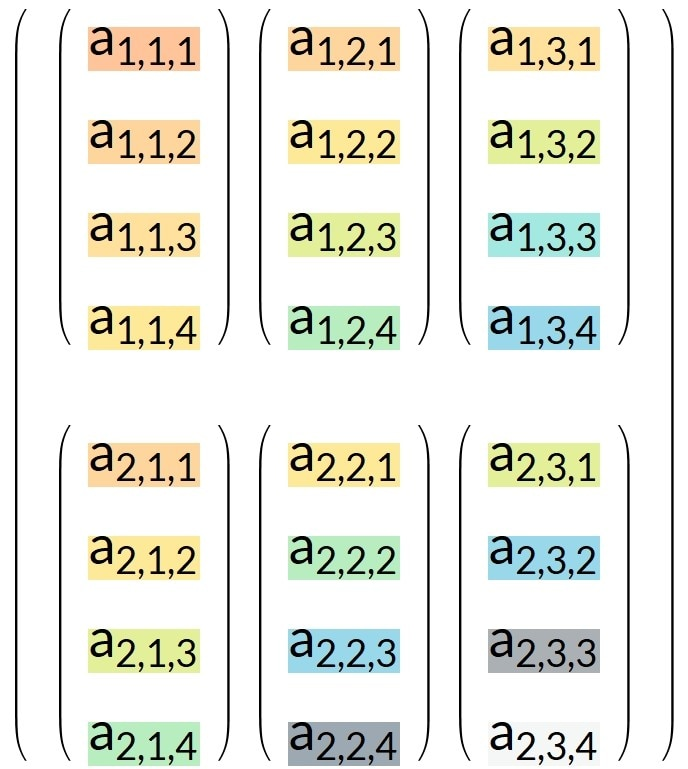This tensor can also be represented as a 3D object, as you can see in the following image: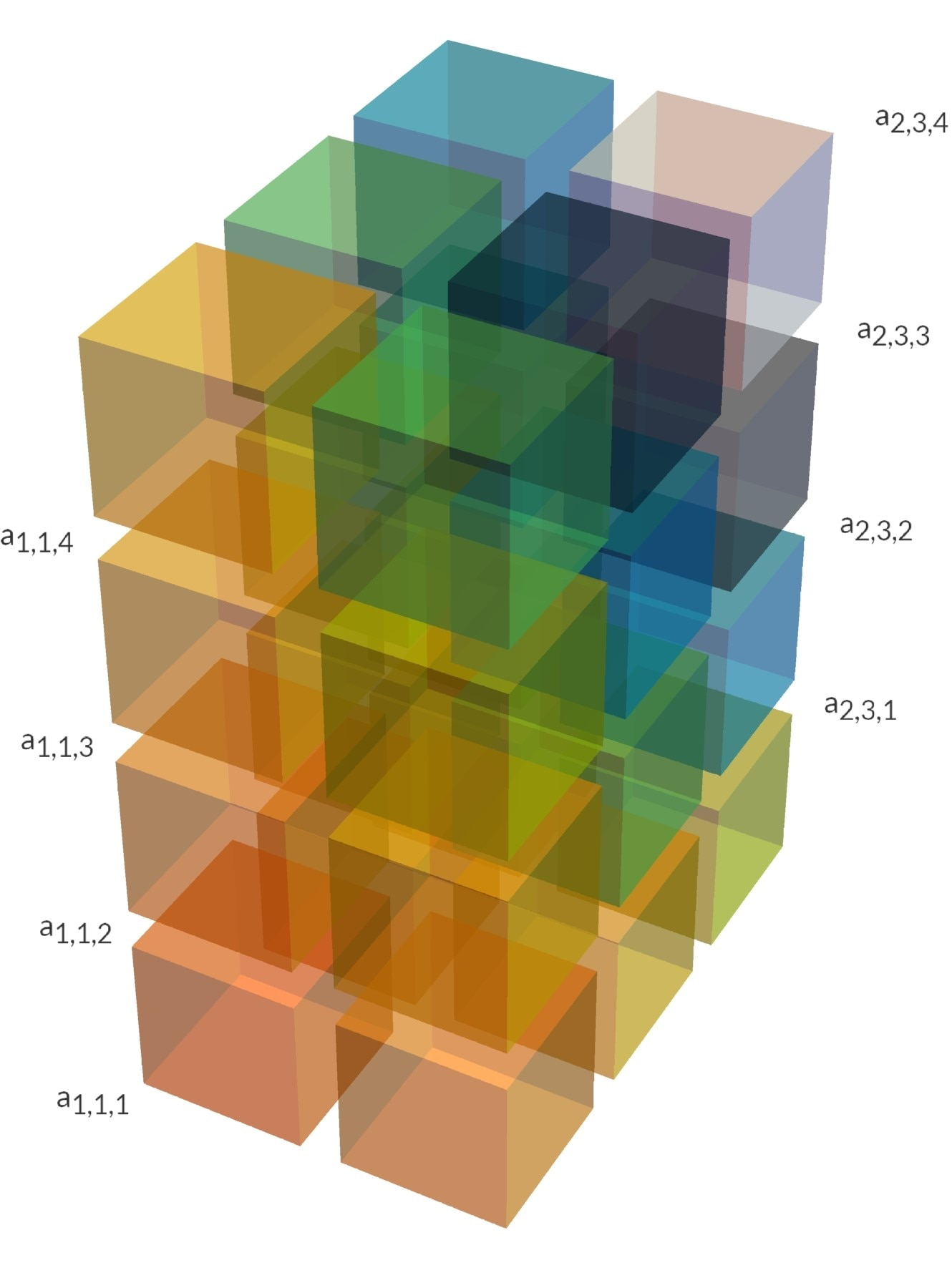The fully connected layer is using a vector as input. It is a flattened version of tensor Torg. Let’s name this flattened tensor FTorg. It is a vector and has also 24 elements, as shown in the following table: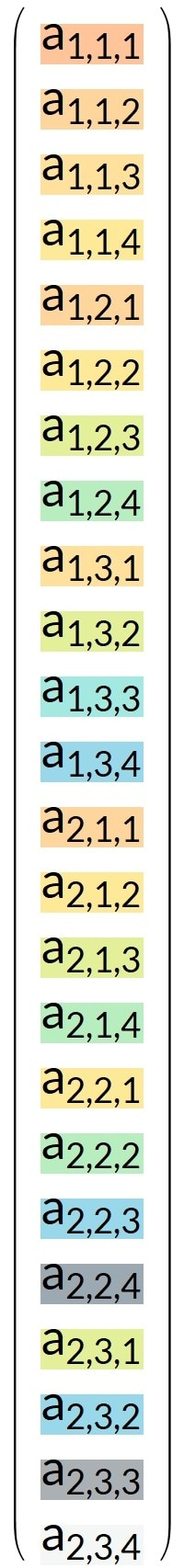Depending on which framework you use, this flattening may, or may not, be modeled in the original ML framework with a flattening layer. Some frameworks do the flattening automatically.

The weight matrix for the original fully connected layer is Worg. Let’s assume that the output of this fully connected layer has two elements, so that the weight matrix has the dimensions {2,24}. The matrix is transforming a vector of 24 elements and into a vector of two elements.

The weight matrix Worg is displayed in the following table: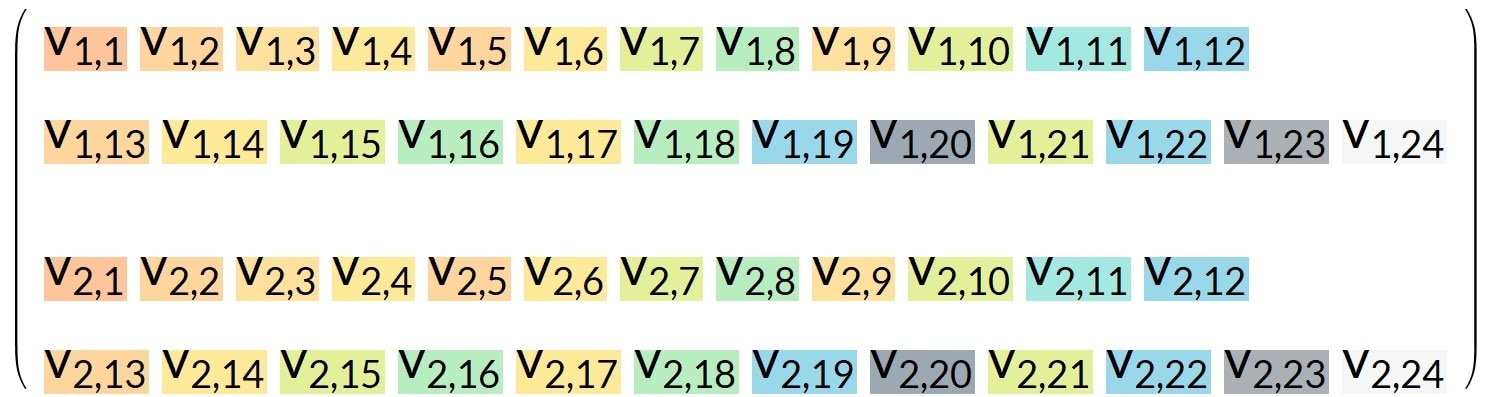The purpose of the fully-connected layer is to compute the matrix product:

Worg. FTorg,

In this example, the fully connected layer output is this column of two values: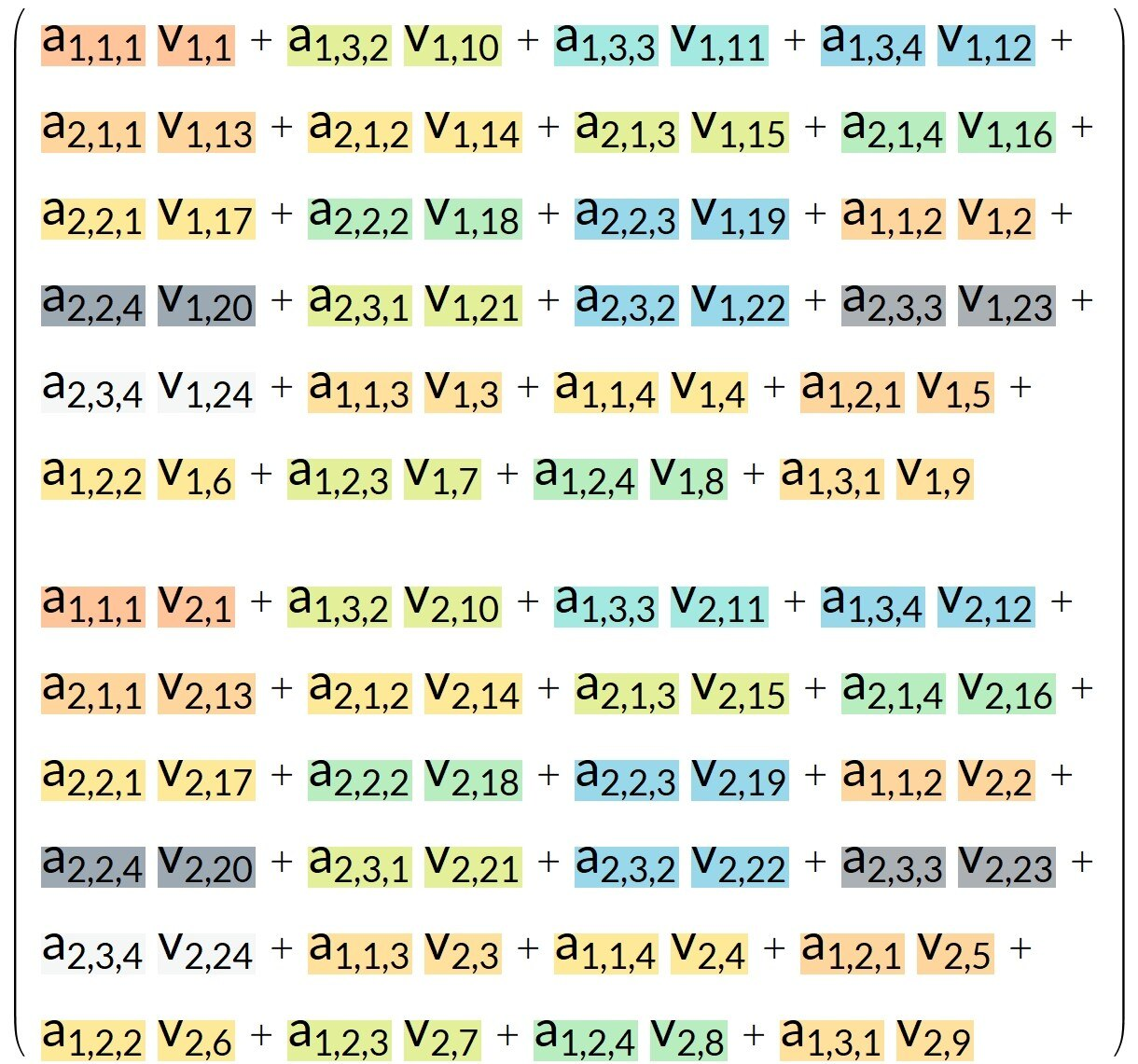Let’s look at an example in which:

• CMSIS-NN orders the data differently to how it is ordered in the original ML framework
• the input tensor is the transposed tensor Tnew with dimensions {4,3,2}

This example is shown in the following table: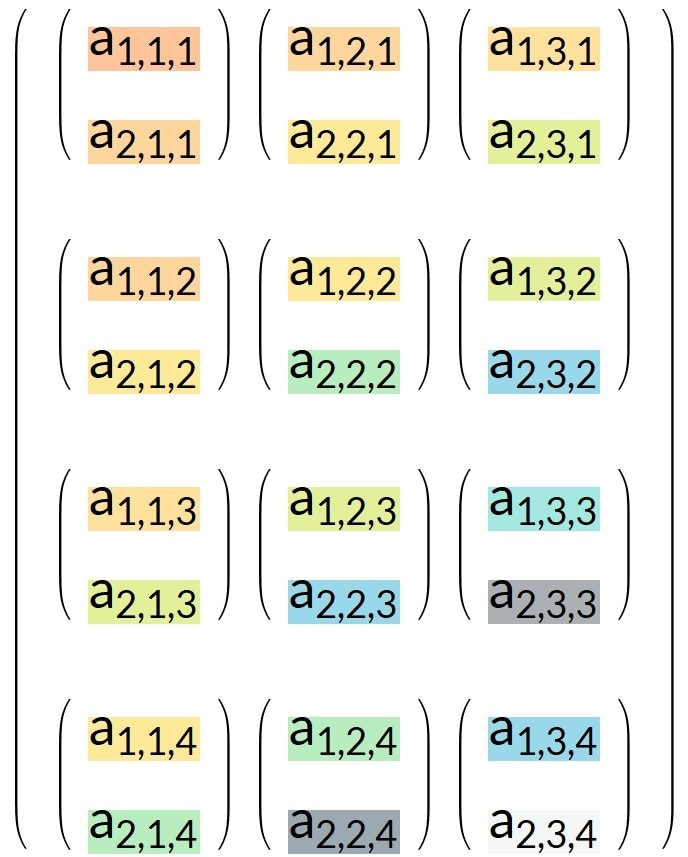This transposed tensor can also be represented as a 3D object, as you can see in this image: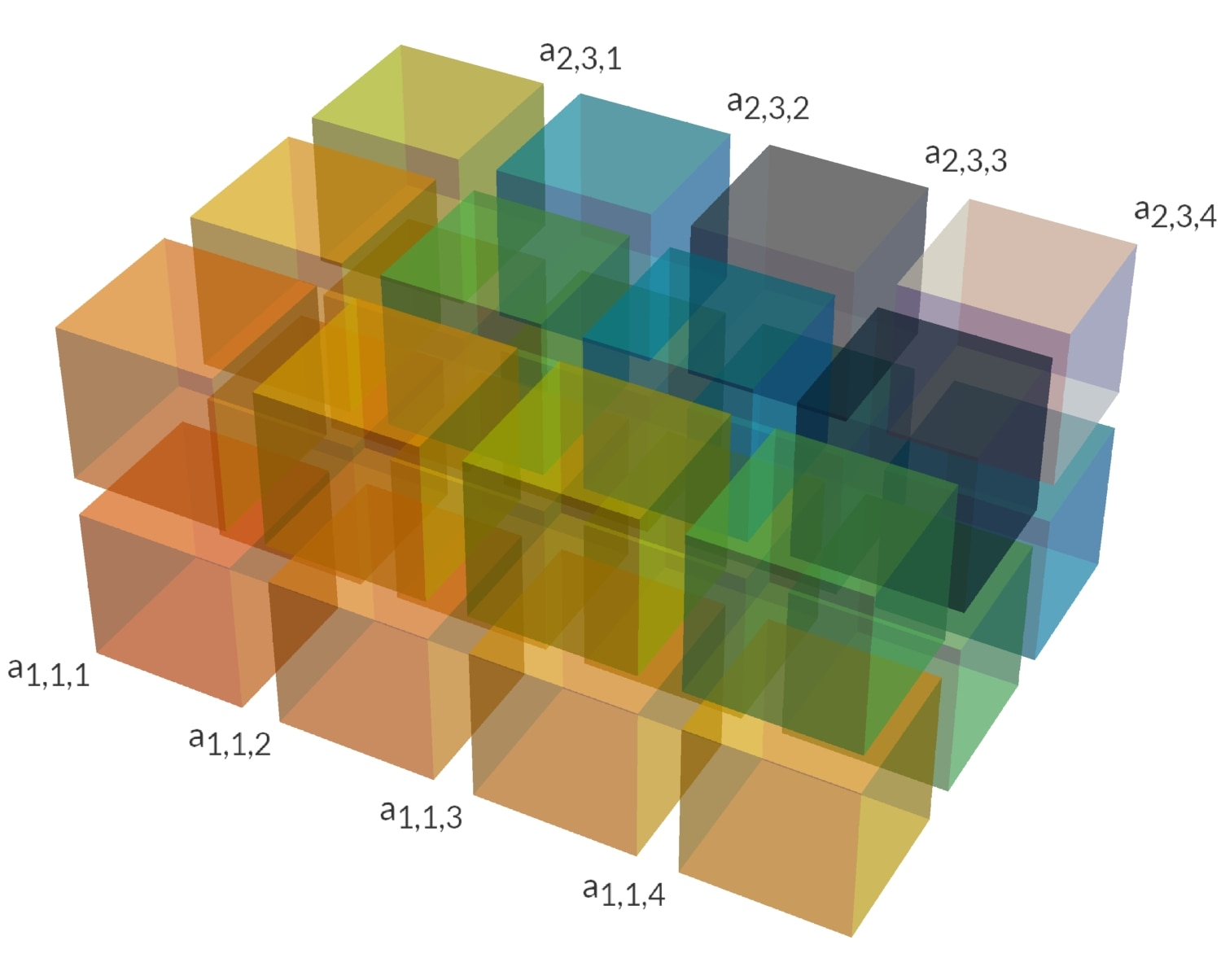Here is an animation showing the transposition operation: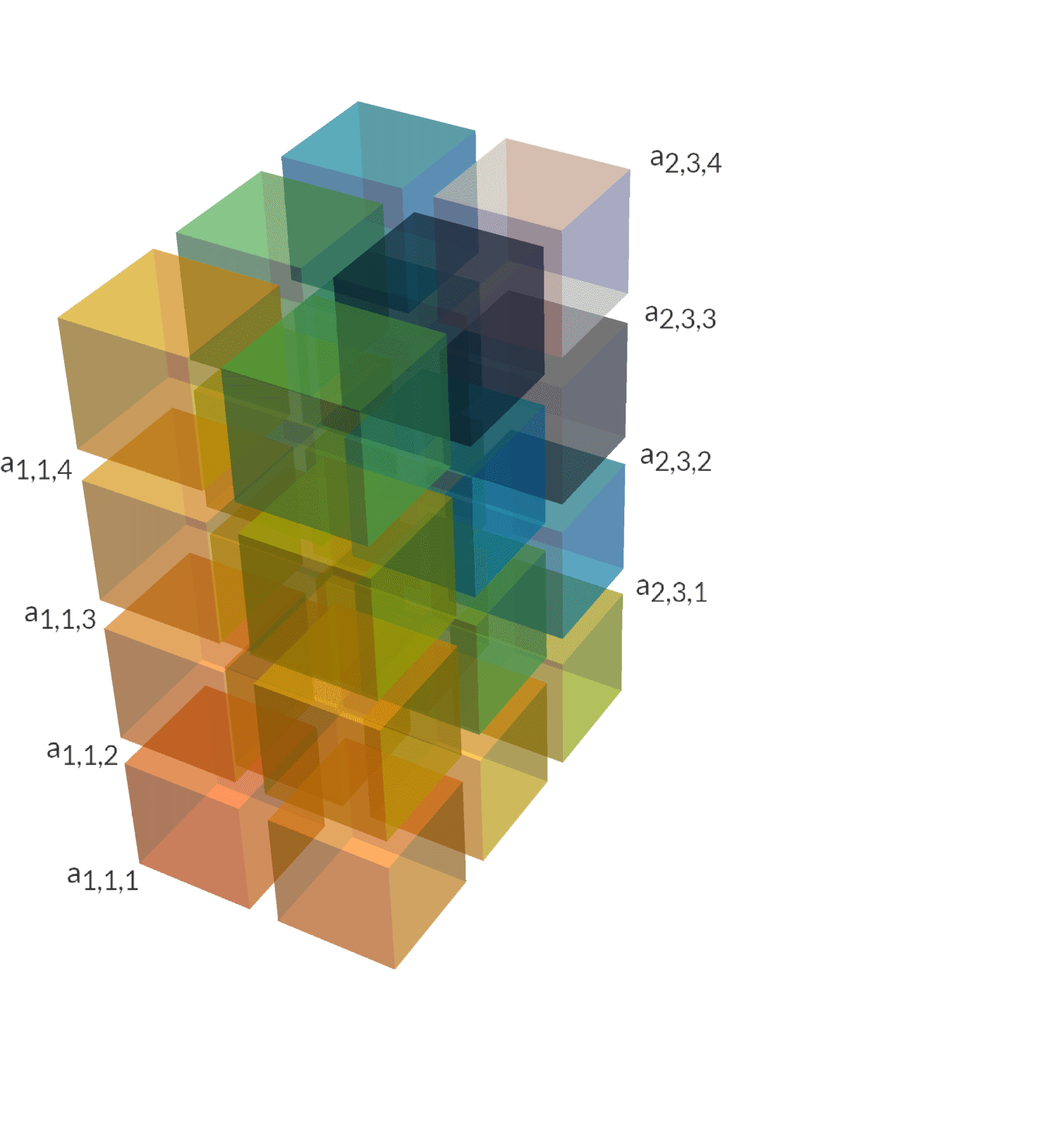In NumPy, if torg is the original tensor, then the conversion can be done with:

tnew = torg.transpose(2,1,0)

The flattened vector FTnew corresponding to tnew is displayed below: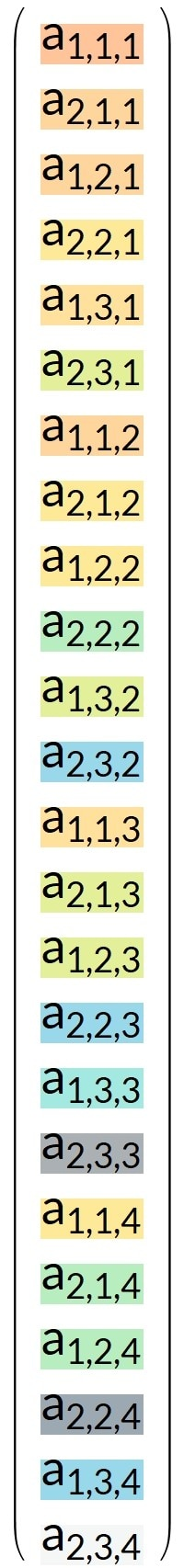In NumPy, this flattened tensor can be computed with:

tnew.reshape(24)

What should the reordering of the weight matrix be, so that the output of the fully connected layer is still the same?

Let’s name this new reordered weight matrix Wnew. For display purposes, here is the transposed version of Wnew.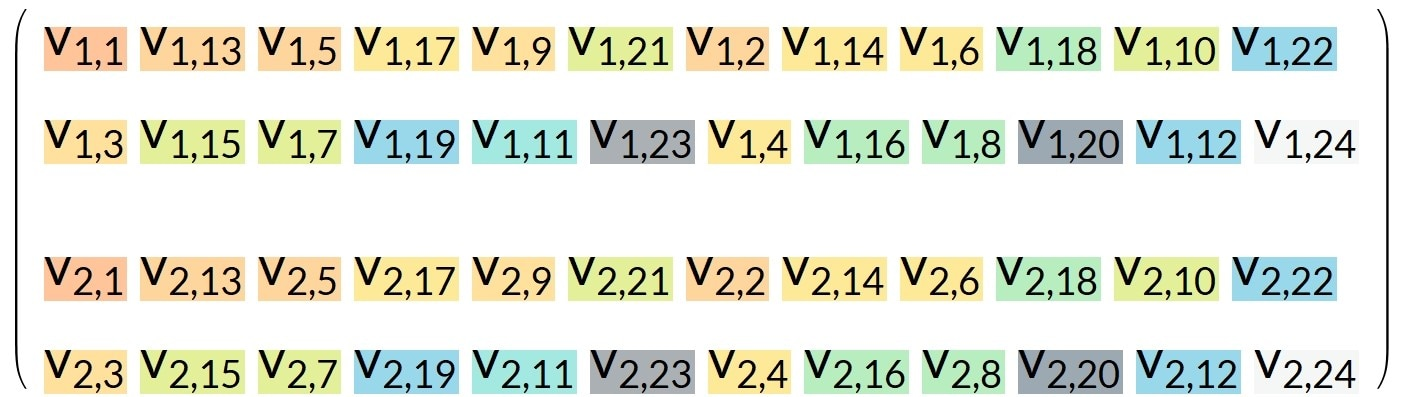Here is an animation showing the weight reordering: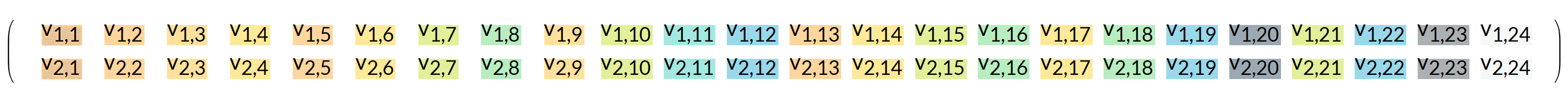If we compute the new matrix product, we must get the same output because the CMSIS-NN implementation must behave as the original ML layer:

Wnew . FTnew == Worg . FTorg

In NumPy, if the weight matrix before reordering is named worg, and the weight matrix after reordering is wnew, then we can get the reordering of the weights with:

wnew = worg.reshape(2,2,3,4).transpose(0,3,2,1).reshape(2,24)

Then we can check that the output computed with reordered weights and reordered input is the same. In NumPy, it can be checked that:

np.dot(worg ,torg.reshape(24)) should be equal to np.dot(wnew , tnew.reshape(24))

The NumPy code for reordering can be decomposed like this:

• The beginning of the line, worg.reshape(2,2,3,4), is transforming the matrix into a tensor. The matrix has dimensions {2,24}. In this example, 2 is the output dimension of the fully connected layer. The input vector of length 24 is the flattened version of a tensor of dimensions {2,3,4} and we need to recover those dimensions. The numbers in the reshape command can be easily related to the input and output dimensions. The command can be written in pseudo-code as: reshape(output dimension, input tensor dimensions).
• Once the weight matrix has been converted into a tensor, it is possible to permute its dimensions. This permutation should correspond to the permutation applied on the input when going from the ML framework to CMSIS-NN. This permutation is the transpose(0,3,2,1) part of the above code. The output dimension is not permuted hence a first 0. Only the tensor dimensions are permuted, and in this example the permutation is a reversal.
• Finally, the tensor is converted back into a {2,24} matrix.
##### Example with a convolutional layer

In case CMSIS-NN is using a different ordering convention than the ML framework, then the procedure to reorder the weights for a convolutional layer is the same as the one for a fully connected layer, but simpler. This is because you do not need to convert a matrix into a tensor and back. The permutation of dimensions can be directly applied to the tensor.

In CMSIS-NN, the input and output of convolutional layers have the following dimensions:

{in channel, x dimension, y dimension}

From the C code for the inner loop of the convolutional layer in CMSIS-NN, we can read that the weight tensor has dimensions:

{in channel, x kernel, y kernel, out channel}

Now, assume your ML framework is working with inputs of dimensions:

{y dimension, x dimension, in channel}

And assume that the weight tensor of the ML framework has corresponding dimensions:

{y kernel, x kernel, in channel, out channel}

The dimensions must be reordered so that the new weight tensor has the dimensions in the order that CMSIS-NN expects:

{in channel, x kernel, y kernel, out channel}

And it is clearly visible that it is just a reverse of the three first dimensions.

In NumPy, it could be done with:

tnew = torg.transpose(2,1,0,3)

The first three dimensions are reversed. The last one is not changed.

Because any necessary reordering is not simple and depends on the details of your ML framework, test the final reordering with a float version of CMSIS-NN. There is no official float version, but it is easy to create one, as we will show

Extract the q15 version from the reference implementations used for the unitary testing of CMSIS-NN. The reference implementations can be found in CMSIS/NN/NN_Lib_Tests/Ref_Implementations.

In the reference q15 implementation:

• q15 must be replaced by float
• The bias and out shifts must be removed
• Saturation functions must be removed

The result will be a float version that can be used to validate the weight reordering, if any reordering is needed.

Note: In CMSIS-NN, there is another reordering of the weights which can be applied for performance purpose, only when the _opt versions of the fully connected functions are used. This weight reordering is explained in the optimization section of this guide.

At the end of this section of the guide, you should know what reordering is required for each layer and how to do it. This reordering will be needed when it is time to dump the coefficients for use with CMSIS-NN.